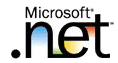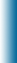Welcome to Mike95.com
 Home Features C++ Library Source Code: COM / ASP Tutorials: Sample Work Internet Based Visual C++ / MFC Java Personal
C++ Templates
Template Function Specialization

In some cases it is possible to override the template-generated code by providing special definitions for specific types. This is called template specialization. The following example demonstrates a situation where overriding the template generated code would be necessary:

```#include <iostream>
using namespace std ;

//max returns the maximum of the two elements of type T,
//where T is a class or data type for which operator>
//is defined.
template <class T>
T max(T a, T b)
{
return a > b ? a : b ;
}

int main()
{
cout << "max(10, 15) = " << max(10, 15) << endl ;
cout << "max('k', 's') = " << max('k', 's') << endl ;
cout << "max(10.1, 15.2) = " << max(10.1, 15.2)
<< endl ;
cout << "max(\"Aladdin\", \"Jasmine\") = "
<< max("Aladdin", "Jasmine") << endl ;
return 0 ;
}
```

### Program Output

```max(10, 15) = 15
max('k', 's') = s
max(10.1, 15.2) = 15.2
```

Not quite the expected results! Why did that happen? The function call max("Aladdin", "Jasmine") causes the compiler to generate code for max(char*, char*), which compares the addresses of the strings! To correct special cases like these or to provide more efficient implementations for certain types, one can use template specializations. The above example can be rewritten with specialization as follows:

```#include <iostream>
#include <cstring>
using namespace std ;

//max returns the maximum of the two elements
template <class T>
T max(T a, T b)
{
return a > b ? a : b ;
}

// Specialization of max for char*
template <>
const char* max(const char* a, const char* b)
{
return strcmp(a, b) > 0 ? a : b ;
}

int main()
{

cout << "max(10, 15) = " << max(10, 15) << endl ;
cout << "max('k', 's') = " << max('k', 's') << endl ;
cout << "max(10.1, 15.2) = " << max(10.1, 15.2)
<< endl ;
cout << "max(\"Aladdin\", \"Jasmine\") = "
<< max("Aladdin", "Jasmine") << endl ;
return 0 ;
}
```

### Program Output

```max(10, 15) = 15
max('k', 's') = s
max(10.1, 15.2) = 15.2